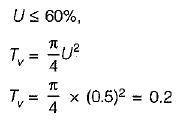Courses

# Test: Compressibility & Consolidation - 2

## 10 Questions MCQ Test GATE Civil Engineering (CE) 2022 Mock Test Series | Test: Compressibility & Consolidation - 2

Description
This mock test of Test: Compressibility & Consolidation - 2 for Civil Engineering (CE) helps you for every Civil Engineering (CE) entrance exam. This contains 10 Multiple Choice Questions for Civil Engineering (CE) Test: Compressibility & Consolidation - 2 (mcq) to study with solutions a complete question bank. The solved questions answers in this Test: Compressibility & Consolidation - 2 quiz give you a good mix of easy questions and tough questions. Civil Engineering (CE) students definitely take this Test: Compressibility & Consolidation - 2 exercise for a better result in the exam. You can find other Test: Compressibility & Consolidation - 2 extra questions, long questions & short questions for Civil Engineering (CE) on EduRev as well by searching above.
QUESTION: 1

### A stratum of sand and a stratum of clay have the same thickness of 3 m. The coefficient of compressibility of sand is 1/5th of coefficient of compressibility of clay and the permeability of sand is 1 0,000 times that of the clay. As suming the same void ratio, the ratio of the consolidation time for the clay to that of the sand is

Solution:

coefficient of consolidation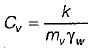using 1 for sand and 2 forclay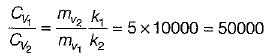As the thickness of both layer is same, so for same degree of consolidation: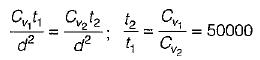QUESTION: 2

### If the time required for 50% consolidation of a remoulded sample of clay with single drainage is t, then the  time required to consolidate the same sample of clay with same degree of consolidation but with double drainage is

Solution: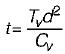For single drainage,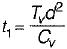For double drainage,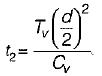⇒ or, T2 = t1/4

QUESTION: 3

### Match List- I and List - II on the basis of Terzaghi's one dimensional consolidation theory and select the  correct answer  using the codes given below the lists (Notations have their usual meaning):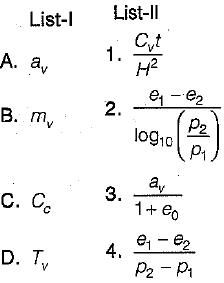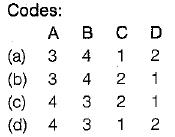Solution:
QUESTION: 4

The unit of the coefficient of consolidation is

Solution:

Coefficient consolidation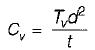Tv is constant and unit less d is drainage path, unit is centimeter (cm) t is time of consolidation , unit is sec.
∴ unit of Cv = cm2/ sec

QUESTION: 5

When the primary consolidation process in a soil is complete, then

Solution:

Only excess pore pressure completely dissipates and effective stresses increase

QUESTION: 6

The ultimate consolidation settlement of a structure resting on a soil

Solution:

ultimate consolidation settlement is given by,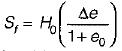so as initial void ration e0 , increase ultimate settlement  decrease.

QUESTION: 7

A normally consolidated clay layer settles by 25 mm when the effective stress is increased from 15 kPa to 30 kPa. If the effective stress is later increased further from 30 kPa to 60 kPa, then the additional settlement would be

Solution: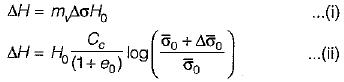These are two equations for calculating the total settlement. The coefficient of volume compressibility (mv) depends up on the stress range and therefore not a constant. However compression index (Cc) is constant for a normally consolidated clay.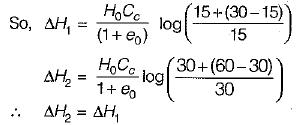= ΔH2 = ΔH1 = 25 mm

QUESTION: 8

Two specimens of clay A and B are tested in a consolidation apparatus.
If (mv)A = 3.6 x 10-4 m2/ kN and
(mv)B = 1.8 x 10-4 m2/kN,
(Cv)A = 3.8 x 10-4 cm2/s,
(Cv)B = 1.9 x 10-4 cm2/s,
then the ratio kA/kB is equal to

Solution: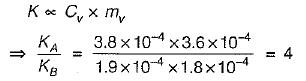QUESTION: 9

In consolidation testing, curve fitting method is used to determine

Solution:

Curve fitting methods like (1) square-root of time method and (2) logarithm of time method are used to determine coefficient of consolidation. The curve between dial gauge reading and time t obtained in the laboratory by testing the soil sample is similar in shape to the theoretical curve between U and Tv obtained from the consolidation theory. This similarity between the laboratory curve and the theoretical curve is used for the determination of the coefficient of consolidation (Cv) of the soil.

QUESTION: 10

When the degree of consolidation is 50% , the time factor is about

Solution:

For